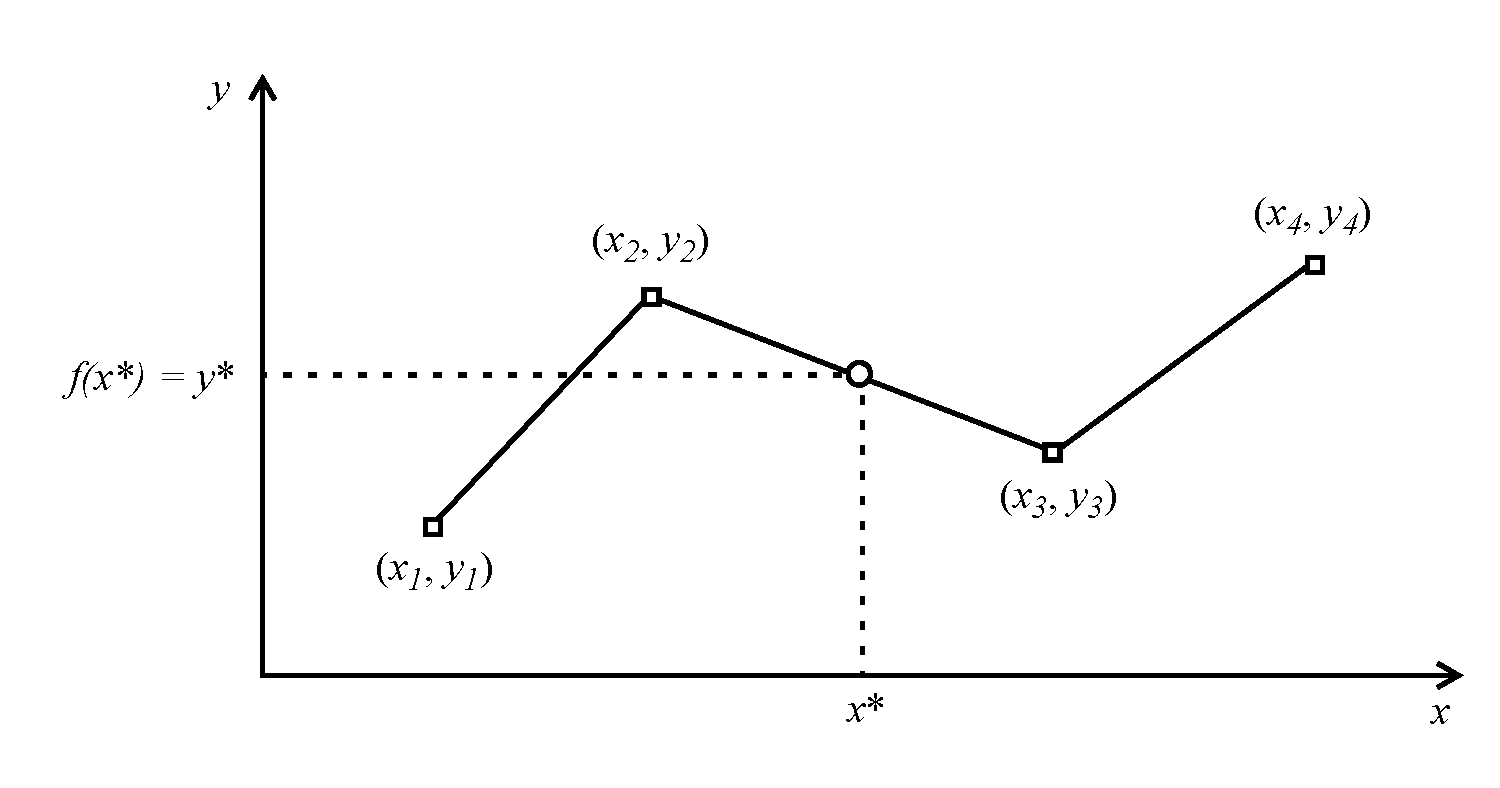Objects and Methods : Table Interpolation : Two-dimensional Table Interpolation
Two-dimensional Table Interpolation
For two-dimensional interpolation, RiverWare assumes that the values in the x column of the data table are increasing. Table B.1 is an example of the proper way to formulate a table for two-dimensional interpolation.

Table B.1  Elevation volume table for a reservoir
Pool Elevation (ft)
Storage (acre-ft)
440
439,400
441
455,900
442
472,600
443
489,600
445
507,000
For two-dimensional functions, we apply linear interpolation between data points. Figure B.1 illustrates this approach. We denote a particular approximation using the table by an asterisk: y* = f(x*).
Figure B.1  Two-dimensional linear interpolationTwo-dimensional Table Interpolation Errors
The following types of errors may be reported during two-dimensional table interpolation:
• Invalid value (data error): an x or y value is invalid (xi = NaN or yi = NaN, for some i).
• Non-increasing x (data error): the x values are not increasing (xi >= xi-1, for some i).
• Out of range (interpolation error): the x value being interpolated is out of the range of the table, that is, the domain of the function being approximated (x* < xmin or x* > xmax).
Revised: 01/11/2023